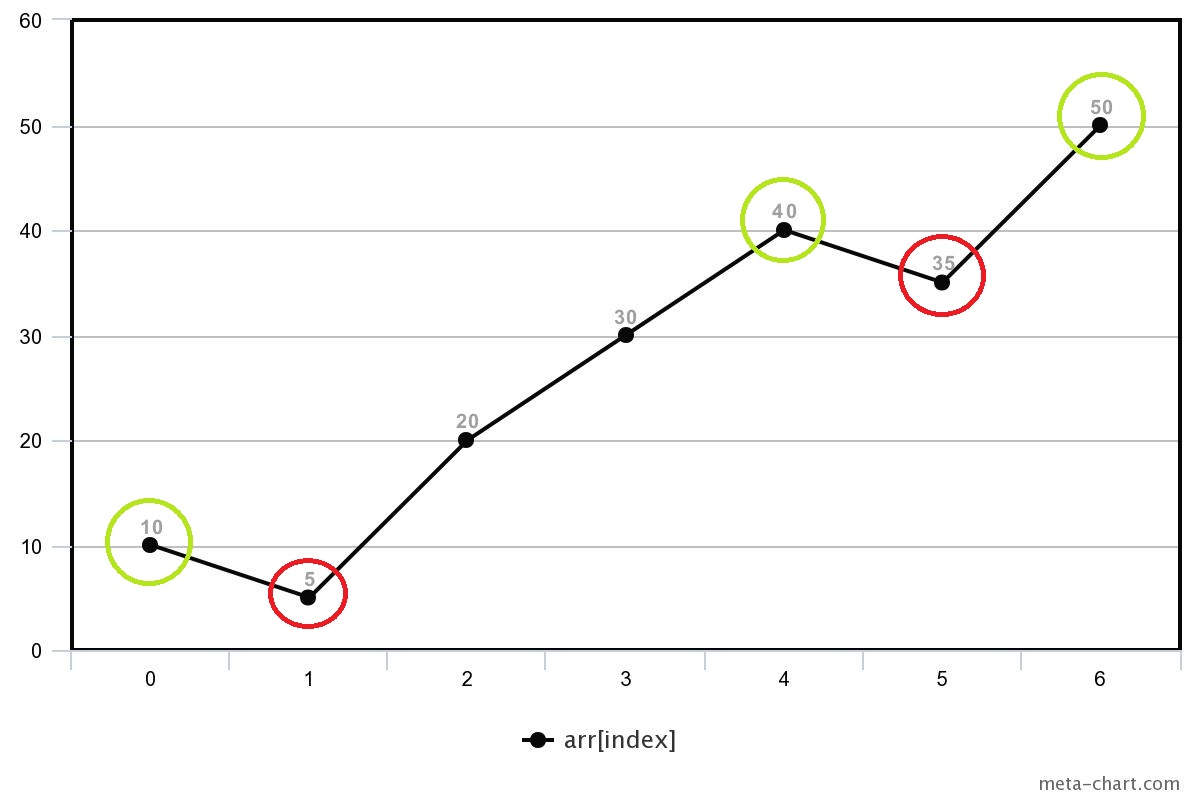# Find Indices For Local Minimas and Maximas

Posted: 12 Nov, 2020
Difficulty: Easy

## PROBLEM STATEMENT

#### We say that an array element arr[i] is a local minimum if it is less than to its neighbours, and local maximum if it is greater than to its neighbours.

##### Note:
``````For corner elements, we need to consider only one neighbour for comparison.
``````
##### For Example:
``````For the given array :  10 5 20 30 40 35 50
````````````The red circle at index 1,5 are local minima because elements at index 1, and 5 both are smaller to its neighbours.

The green circle at index 0,4,6 are local maxima, because element at index 4 is greater than its neighbours, and element at 0, and 4 are corner elements and also greater than its one neighbour.
``````
##### Input format :
``````The first line of input contains an integer 'T' representing the number of test cases or queries to be processed.

Then the test case follows.

The first line of each test case contains integer N denoting the size of the array.

The second line of each test case contains 'N' single space-separated integers representing the array elements.
``````
##### Output Format:
``````For each test case
In the first line, print all the space-separated indices of local minima in the given array

In the second line, print all the space-separated indices of local maxima in the given array.
``````
##### Note:
``````You do not need to print anything, it has already been taken care of. Just implement the given function.
``````
##### Constraints:
``````1 <= T <= 10
2 <= N <= 10^5
-10^9 <= arr[i] <= 10^9

Time Limit: 1 sec
``````Approach 1

The idea is to iterate through the given array and at each index i, we check its neighbors to check if it's local minima or maxima.

Steps:

• We create two lists namely minima and maxima, to store all the indices of local minima and local maxima respectively.
• First, we check for the local maxima and minima conditions for the first element.
• Then we iterate through the given array from index 1 to N - 2, and at any index i, we do the following:
• If arr[i – 1] > arr[i] < arr[i + 1] then add index i to minima.
• If arr[i – 1] < arr[i] > arr[i + 1] then add index i to maxima.
• Then, we check for the local maxima and minima conditions for the last element.